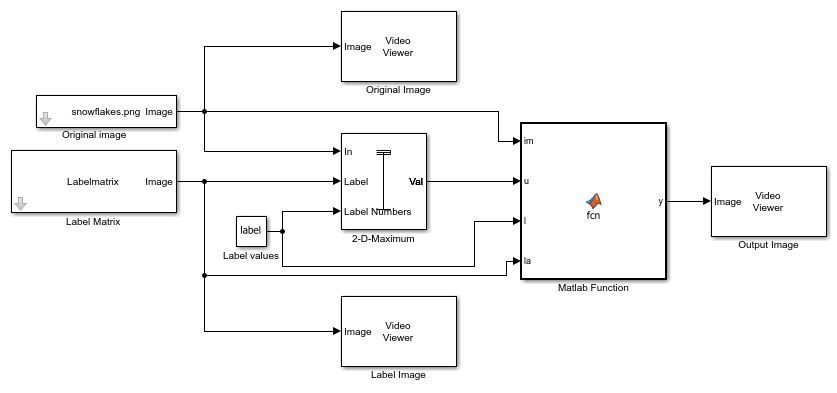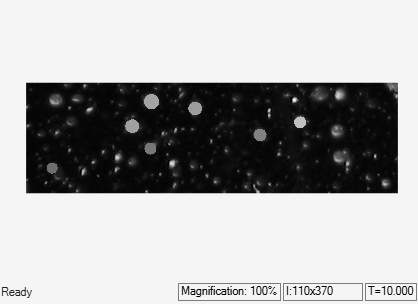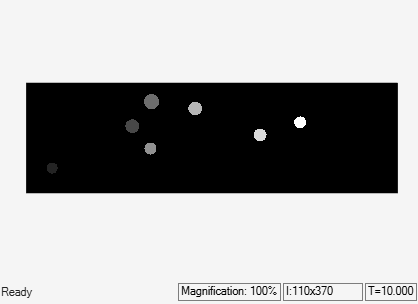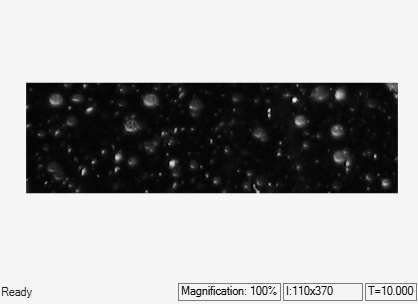Main Content

# Replace Intensity Values in ROI with its Maximum Value

This example shows how to find maximum intensity value of region of interests (ROI) in the input image and replace the pixels in the ROI with its maximum value

### Load Data to MATLAB Workspace

The input to the model is the original image, label matrix, and the label values. The label matrix contain the desired ROIs in the input image. Load the label matrix into MATLAB workspace.

```load Snowflakes_mask.mat ```

Find the unique label values in the label matrix. The label value 0 corresponds to the background and must be ignored.

```lb = unique(Labelmatrix); label = lb(2:end); ```

### Example Model

Open the Simulink model. The model reads the input image using the `Image` `From` `File` block.

```open_system('ex_blk2dmaximum.slx'); ```The model computes the maximum intensity value for each ROI and replaces all the pixel values in the ROI to maximum value. The model computes the maximum value for each ROI individually by setting these `2-D Maximum` block parameters to the specified value,

• Set the `Mode` parameter to `Value`.

• Set the `Find the maximum value over` parameter to `Entire` `input`.

• Set the `Enable ROI processing` parameter and set the `ROI` `type` parameter as `Label` `matrix`.

The `Matlab` `Function` block replaces the individual ROI's with its maximum intensity value and outputs the resultant image.

### Simulate and Display Results

Run the model and display the images using `Video` `Viewer` block.

```sim('ex_blk2dmaximum.slx'); ```Download ebook